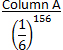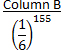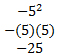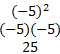Quantity Comparison Practice Questions

For questions 1-10, compare the quantities in Columns A and B.

1. Use the system of equations below to compare Columns A and B.

x + 2y = 1
x + y = 5

 Column A Column B –x y
1. The quantity in Column A is greater.
2. The quantity in Column B is greater.
3. The two quantities are equal.
4. It is impossible to determine which quantity is greater.

2.

 Column A Column B The average of 4, 5, 8, 12, and 13 The average of 4, 5, 8, and 12
1. The quantity in Column A is greater.
2. The quantity in Column B is greater.
3. The two quantities are equal.
4. It is impossible to determine which quantity is greater.

3.

 Column A Column B The perimeter of a rectangle whose area is 20 The area of a rectangle whose perimeter is 20
1. The quantity in Column A is greater.
2. The quantity in Column B is greater.
3. The two quantities are equal.
4. It is impossible to determine which quantity is greater.

4.

 Column A Column B 35% of 60 60% of 35
1. The quantity in Column A is greater.
2. The quantity in Column B is greater.
3. The two quantities are equal.
4. It is impossible to determine which quantity is greater.

5.

 Column A Column B -52 (-5)2
1. The quantity in Column A is greater.
2. The quantity in Column B is greater.
3. The two quantities are equal.
4. It is impossible to determine which quantity is greater.

6.1. The quantity in Column A is greater.
2. The quantity in Column B is greater.
3. The two quantities are equal.
4. It is impossible to determine which quantity is greater.

7. The price of an item was increased by 20%. A week later, its new price was discounted by 20%.

 Column A Column B The original price of the item The final price of the item
1. The quantity in Column A is greater.
2. The quantity in Column B is greater.
3. The two quantities are equal.
4. It is impossible to determine which quantity is greater.

8.

 Column A Column B y3 y-2
1. The quantity in Column A is greater.
2. The quantity in Column B is greater.
3. The two quantities are equal.
4. It is impossible to determine which quantity is greater.

9.

 Column A Column B one-sixth of 72 one-fifth of 65
1. The quantity in Column A is greater.
2. The quantity in Column B is greater.
3. The two quantities are equal.
4. It is impossible to determine which quantity is greater.

10. Use the figure below to compare Columns A and B.Column A Column B x 38
1. The quantity in Column A is greater.
2. The quantity in Column B is greater.
3. The two quantities are equal.
4. It is impossible to determine which quantity is greater.

1. A. To compare the quantities in Columns A and B, first solve the system of equations for x and y. Since one equation has x and the other has –x, eliminate x by adding the equations together.To find the value of x, substitute 2 for y in the first equation and solve.Therefore, the solution of the given system of equations is x = -3,y = – 2. Now compare the values of Columns A and B. The value of Column A is –x, which is 3, and the value of Column B is y, which is -2. Thus, Column A is greater.

2. A. The formula for the average of a list of terms is shown below.One way to solve the problem is to use this formula to calculate the averages of each list of numbers. Another way to compare Columns A and B is to notice that both columns contain the numbers 4, 5, 8, and 12, but only Column A contains the number 13. Since 13 is higher than the other numbers, its inclusion causes the average to increase.  Therefore, the average of the numbers in Column A is greater.

3. D. One way to compare the two quantities is to think of some of the different values that rectangles’ lengths and widths could take. For instance, the dimensions of the rectangle in Column A could be 1 x 20, giving it a perimeter of 42; an alternative rectangle’s dimensions could be 4 x 5, giving it a perimeter of 18.

The dimensions of the rectangle in Column B could be 1 x 9, giving it an area of 9an alternative rectangle’s dimensions could be 5 x 5, giving it an area of 25.

So, if Column A is 42 and Column B is 9, then Column A is greater. But if Column A is 18 and Column B is 25, then Column B is greater. Therefore, it is impossible to determine which quantity is greater

4. C. Calculate the values of Column A and Column B. Recall that the word “of” means multiplication.

 Column A Column B 35% of 60 0.36∙60 21 60% of 35 0.60∙35 21

Notice that the two quantities are equal.

5. B. Calculate the values of each column. When the minus sign is not inside parentheses, it is applied after the exponent is simplified. When the minus sign is inside parentheses, it is part the negative number is the base of the exponent.

 Column A Column BNotice that the quantity in Column B is greater.

6. B. It would be very hard to calculate eitheroras decimals, so use reasoning to determine which is greater. Notice that both exponential expressions have the same base, and there exponents differ by just one. Column A can be rewritten to make its comparison with Column B easier.Sinceis a positive number, multiplying it bywill make it smaller. In other words, the quantity in Column B is greater.

7. A. Although it is not necessary, this problem will be easier to solve if you choose a value for the original price of the item. Say, for example, the original price was \$100. Since 20% of \$100 is \$20, the price of the item became \$120. Next, the price was discounted by 20%; since 20% of 120 is 24, the final price was \$96. The original price of \$100 is greater than the final price of \$96, so the quantity in Column A is greater.

8. D. By definition,. Thus, y-2 is equivalent to. Because the value of y is unknown, however, it’s not possible to determine whether y3 is greater or lesser than y2. For instance, if y = 5, then Column A is greater. But if, then Column B is greater. Therefore, it is impossible to determine which quantity is greater.

9. B. Calculate the values of Column A and Column B. Recall that the word “of” means multiplication.Notice that the quantity in Column B is greater.

10. B. The sum of the angles of a triangle is 180°. Notice that the top angle is a right angle, so it is 90°; the other given angle measures 62°. To determine the measure of x, subtract the given angle measurements from 180°.

180 – 90 – 62 = 28

Therefore, the missing angle is 28°, so the quantity in Column B is greater.

Last Updated: June 4, 2019